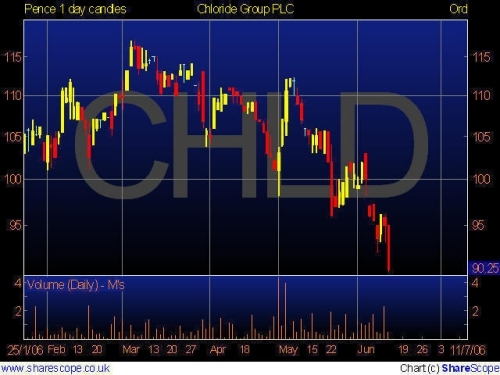# Using Pivot Points to make Better Trades

Pivot Points have been used by floortraders at the major equities and futures exchanges for a long time. Tradersfound that the price tended to hover near the pivot level and trade in betweenthe pivot and support and resistance levels generated by a simple calculationbased on the previous day?s high low and close. One advantage of using Pivotsis they are a predictive indicator as opposed to lagging.

Predictive Vs Lagging Indicators

Classic Pivot Points

To calculate the pivot point, take theprevious day or session?s high(H), low(L) and close(C), add them together anddivide by 3 [(H+L+C/3 = Pivot Point (PP)] Now we can calculate the support andresistance levels based offthe pivot,

S1 = (PP x 2)-H R1 = (PP x 2) ? L S2 = (PP-Range) S3 = (PP-Range) x 2 S4 = (PP-Range)x 3 R2 = (PP+Range) R3 = (PP+Range x 2) R4 = (PP+Range x 3)Range =High-Low.

E-mini S&P500 Chart:For this example we will use the E-miniS&P 500 futures.

The previous session high = 1124.25,The low = 1110.25Close was 1122.25

Using these values we can calculate the pivotpoint, PP = 1118.92S1 = 1113.57 R1 =1127.58 S2 = 1104.94S3 = 1090.92S4 = 10796.92 R2 = 1132.92 R3 = 1146.92R4 = 1160.92.

Looking at this chart, we see that whenprice fell, it fell exactly to the first level of support and bounced higher.We used the exchange hours to set our session and ignored the Globex/overnightsession when calculcating our pivot points. The reason we did this is becausemuch more volume is traded during the exchange session and the institutionsthat really move the price are trading during these hours. Most institutions donot trade the low liquidity overnight session unless there is a news event. Sowhen the market opened the next day it immediately fell and found supportexactly at S1. The difference between R1 and S1 was about 14 points, which is apretty large range for this contract. In other words, it would take a majorevent to push the price to the next level of support or resistance (S2, R2). Byusing S1 as the buy entry and our pivot point as a take profit level we can useS2 as the Stop Loss level, the difference between S1 and the PP was 5.34 (5.25rounded down, which is 21 ticks on this contract), so that was our profitpotential on this setup. Conversely, we could have set a buy stop slightlyhigher than R1 and a sell stop right below S1, this would be more of a breakoutstrategy where we look for the price to move through either level with momentumand continue down to S2 or move higher to R2.

Alternate Calculation Methods

There are several methods to calculatepivot points, 2 alternate methods are Woodie?s and Fibonacci. For Woodie?smethod instead of using the previous session?s closing price, we use the currentsession?s open price.The formula to calculate the pivot point is

PP = (H+L+(Today?sOpenx2)/4.

Our Support and Resistance levels are calculated the same way as theclassic method. Another method is Fibonacci, this method uses the Classiccalculation to find the Pivot Point of (H+L+C)/3 but uses the major fibonaccilevels to calculate support and resistance levels.

S1 = PP-0.382 x (H-L)S2 = PP-0.618 x (H-L)S3 = PP-1.0 x (H-L) R1 = PP+0.382 x (H-L) R2 = PP+0.618 x (H-L)R3 = PP+1.0 x (H-L)

Here is a table using the same Open, High ,Low and Close data to compare the different calculation methods:Combining Pivots with other tools.

Pivot Points are a valuable tool for any trader,however, no single tool tells the whole story, we are looking for multiple indicatorsto align and confirm a move. Meaning, if the price is nearing R1, our RSI isabove 90 and we have an important Fibonnacci level at or around the same price,that validates our prediction that we will encounter resistance more thanrelying on any 1 indicator. It is important to look at different indicators thattell a different story. For example if you are using a moving average crossoverfor entry confirmed by a MACD, you are basically looking at the same thing intwo different ways, a MACD measures the difference between 2 moving averages soof course the signal will be confirmed! But if we mix the inidcators up byusing a momentum indicator such as RSI or Stochastics, now we are looking at 2different instruments that are giving a similar reading. Combine theseindicators with support and resistance tools such as pivots and Fibonnacci, nowyou have 3 totally different indicators for confirmation, that means you have amuch better chance of making a good trade. You may find yourself taking lesstrades, however this is about quality, not quanitity. In the end, trading isall about probablilty, and if you put the probability in your favor over andover again, you will increase your chances of coming out on top.

Jesse Richards can be contacted at Fast Trading Services LLC